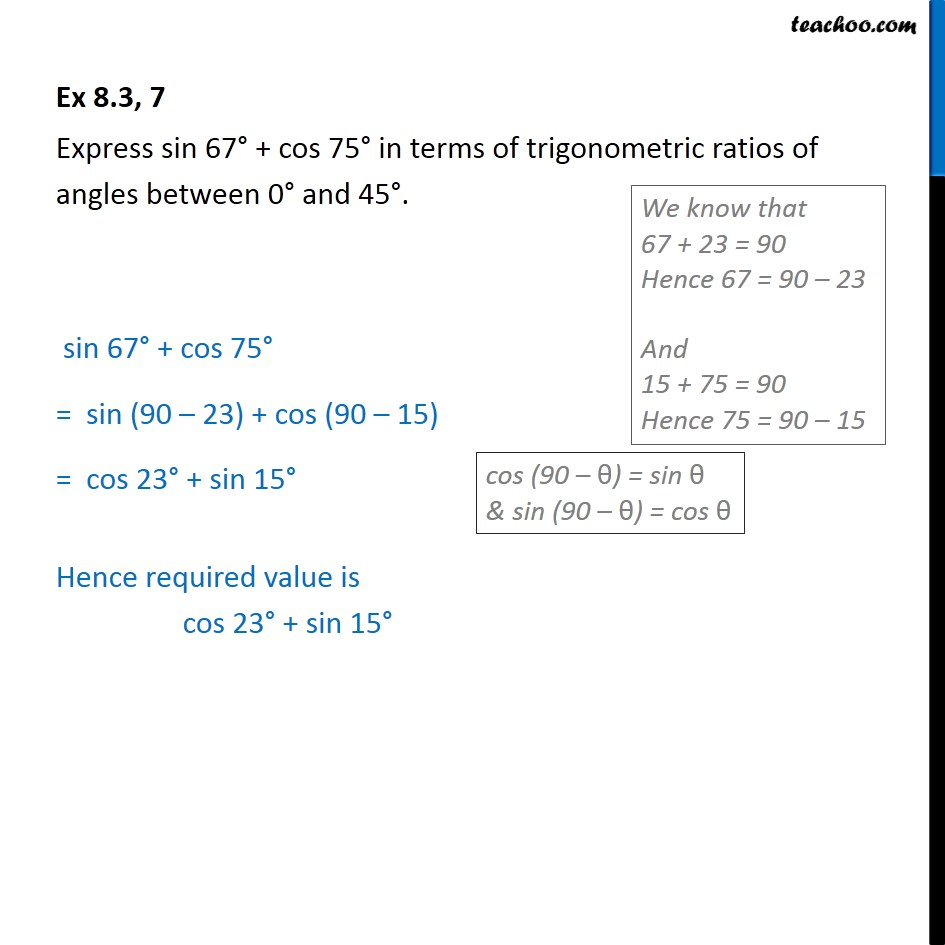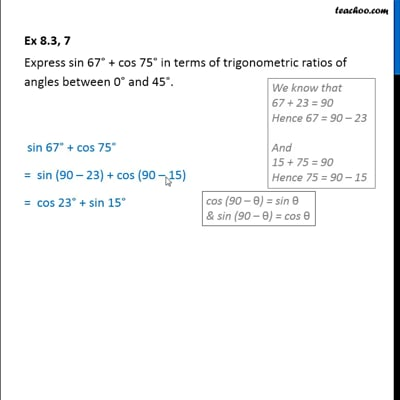Sin 90 - θ, cos 90 - θ formula

Chapter 8 Class 10 Introduction to Trignometry
Serial order wiseThis video is only available for Teachoo black users

Learn in your speed, with individual attention - Teachoo Maths 1-on-1 Class

### Transcript

Question 7 Express sin 67 + cos 75 in terms of trigonometric ratios of angles between 0 and 45 . sin 67 + cos 75 = sin (90 23) + cos (90 15) = cos 23 + sin 15 Hence required value is cos 23 + sin 15